xCentroidOverview

In geometry
Geometry
Geometry arose as the field of knowledge dealing with spatial relationships. Geometry was one of the two fields of pre-modern mathematics, the other being the study of numbers ....

, the centroid, geometric center, or barycenter of a plane figure or two-dimensional shape X is the intersection of all straight lines that divide X into two parts of equal moment
Moment (mathematics)
In mathematics, a moment is, loosely speaking, a quantitative measure of the shape of a set of points. The "second moment", for example, is widely used and measures the "width" of a set of points in one dimension or in higher dimensions measures the shape of a cloud of points as it could be fit by...

about the line. Informally, it is the "average" (arithmetic mean
Arithmetic mean
In mathematics and statistics, the arithmetic mean, often referred to as simply the mean or average when the context is clear, is a method to derive the central tendency of a sample space...

) of all points of X. The definition extends to any object X in n-dimension
Dimension
In physics and mathematics, the dimension of a space or object is informally defined as the minimum number of coordinates needed to specify any point within it. Thus a line has a dimension of one because only one coordinate is needed to specify a point on it...

al space: its centroid is the intersection of all hyperplane
Hyperplane
A hyperplane is a concept in geometry. It is a generalization of the plane into a different number of dimensions.A hyperplane of an n-dimensional space is a flat subset with dimension n − 1...

s that divide X into two parts of equal moment.

In physics
Physics
Physics is a natural science that involves the study of matter and its motion through spacetime, along with related concepts such as energy and force. More broadly, it is the general analysis of nature, conducted in order to understand how the universe behaves.Physics is one of the oldest academic...

, the word centroid means the geometric center of the object's shape, as above, but barycenter may also mean its physical center of mass
Center of mass
In physics, the center of mass or barycenter of a system is the average location of all of its mass. In the case of a rigid body, the position of the center of mass is fixed in relation to the body...

or the center of gravity
Center of gravity
In physics, a center of gravity of a material body is a point that may be used for a summary description of gravitational interactions. In a uniform gravitational field, the center of mass serves as the center of gravity...

, depending on the context.Unanswered QuestionsProperty of centroidsEncyclopedia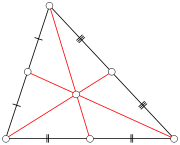In geometry
Geometry
Geometry arose as the field of knowledge dealing with spatial relationships. Geometry was one of the two fields of pre-modern mathematics, the other being the study of numbers ....

, the centroid, geometric center, or barycenter of a plane figure or two-dimensional shape X is the intersection of all straight lines that divide X into two parts of equal moment
Moment (mathematics)
In mathematics, a moment is, loosely speaking, a quantitative measure of the shape of a set of points. The "second moment", for example, is widely used and measures the "width" of a set of points in one dimension or in higher dimensions measures the shape of a cloud of points as it could be fit by...

about the line. Informally, it is the "average" (arithmetic mean
Arithmetic mean
In mathematics and statistics, the arithmetic mean, often referred to as simply the mean or average when the context is clear, is a method to derive the central tendency of a sample space...

) of all points of X. The definition extends to any object X in n-dimension
Dimension
In physics and mathematics, the dimension of a space or object is informally defined as the minimum number of coordinates needed to specify any point within it. Thus a line has a dimension of one because only one coordinate is needed to specify a point on it...

al space: its centroid is the intersection of all hyperplane
Hyperplane
A hyperplane is a concept in geometry. It is a generalization of the plane into a different number of dimensions.A hyperplane of an n-dimensional space is a flat subset with dimension n − 1...

s that divide X into two parts of equal moment.

In physics
Physics
Physics is a natural science that involves the study of matter and its motion through spacetime, along with related concepts such as energy and force. More broadly, it is the general analysis of nature, conducted in order to understand how the universe behaves.Physics is one of the oldest academic...

, the word centroid means the geometric center of the object's shape, as above, but barycenter may also mean its physical center of mass
Center of mass
In physics, the center of mass or barycenter of a system is the average location of all of its mass. In the case of a rigid body, the position of the center of mass is fixed in relation to the body...

or the center of gravity
Center of gravity
In physics, a center of gravity of a material body is a point that may be used for a summary description of gravitational interactions. In a uniform gravitational field, the center of mass serves as the center of gravity...

, depending on the context. Informally, the center of mass (and center of gravity in a uniform gravitational field) is the average of all points, weighted by the local density
Density
The mass density or density of a material is defined as its mass per unit volume. The symbol most often used for density is ρ . In some cases , density is also defined as its weight per unit volume; although, this quantity is more properly called specific weight...

or specific weight
Specific weight
The specific weight is the weight per unit volume of a material. The symbol of specific weight is γ ....

. If a physical object has uniform density
Density
The mass density or density of a material is defined as its mass per unit volume. The symbol most often used for density is ρ . In some cases , density is also defined as its weight per unit volume; although, this quantity is more properly called specific weight...

, then its center of mass
Center of mass
In physics, the center of mass or barycenter of a system is the average location of all of its mass. In the case of a rigid body, the position of the center of mass is fixed in relation to the body...

is the same as the centroid of its shape.

In geography, the centroid of a region of the Earth's surface, projected radially onto said surface, is known as its geographical center.

## Properties

The geometric centroid of a convex
Convex set
In Euclidean space, an object is convex if for every pair of points within the object, every point on the straight line segment that joins them is also within the object...

object always lies in the object. A non-convex object might have a centroid that is outside the figure itself. The centroid of a ring
Annulus (mathematics)
In mathematics, an annulus is a ring-shaped geometric figure, or more generally, a term used to name a ring-shaped object. Or, it is the area between two concentric circles...

or a bowl
Bowl (vessel)
A bowl is a common open-top container used in many cultures to serve food, and is also used for drinking and storing other items. They are typically small and shallow, although some, such as punch bowls and salad bowls, are larger and often intended to serve many people.Bowls have existed for...

, for example, lies in the object's central void.

If the centroid is defined, it is a fixed point of all isometries
Fixed points of isometry groups in Euclidean space
A fixed point of an isometry group is a point that is a fixed point for every isometry in the group. For any isometry group in Euclidean space the set of fixed points is either empty or an affine space....

in its symmetry group
Symmetry group
The symmetry group of an object is the group of all isometries under which it is invariant with composition as the operation...

. In particular, the geometric centroid of an object lies in the intersection of all its hyperplane
Hyperplane
A hyperplane is a concept in geometry. It is a generalization of the plane into a different number of dimensions.A hyperplane of an n-dimensional space is a flat subset with dimension n − 1...

s of symmetry
Symmetry
Symmetry generally conveys two primary meanings. The first is an imprecise sense of harmonious or aesthetically pleasing proportionality and balance; such that it reflects beauty or perfection...

. The centroid of many figures (regular polygon
Regular polygon
A regular polygon is a polygon that is equiangular and equilateral . Regular polygons may be convex or star.-General properties:...

, regular polyhedron
Regular polyhedron
A regular polyhedron is a polyhedron whose faces are congruent regular polygons which are assembled in the same way around each vertex. A regular polyhedron is highly symmetrical, being all of edge-transitive, vertex-transitive and face-transitive - i.e. it is transitive on its flags...

, cylinder
Cylinder (geometry)
A cylinder is one of the most basic curvilinear geometric shapes, the surface formed by the points at a fixed distance from a given line segment, the axis of the cylinder. The solid enclosed by this surface and by two planes perpendicular to the axis is also called a cylinder...

, rectangle
Rectangle
In Euclidean plane geometry, a rectangle is any quadrilateral with four right angles. The term "oblong" is occasionally used to refer to a non-square rectangle...

, rhombus
Rhombus
In Euclidean geometry, a rhombus or rhomb is a convex quadrilateral whose four sides all have the same length. The rhombus is often called a diamond, after the diamonds suit in playing cards, or a lozenge, though the latter sometimes refers specifically to a rhombus with a 45° angle.Every...

, circle, sphere, ellipse
Ellipse
In geometry, an ellipse is a plane curve that results from the intersection of a cone by a plane in a way that produces a closed curve. Circles are special cases of ellipses, obtained when the cutting plane is orthogonal to the cone's axis...

, ellipsoid, superellipse, superellipsoid
Superellipsoid
In mathematics, a super-ellipsoid or superellipsoid is a solid whose horizontal sections are super-ellipses with the same exponent r, and whose vertical sections through the center are super-ellipses with the same exponent t....

, etc.) can be determined by this principle alone.

In particular, the centroid of a parallelogram
Parallelogram
In Euclidean geometry, a parallelogram is a convex quadrilateral with two pairs of parallel sides. The opposite or facing sides of a parallelogram are of equal length and the opposite angles of a parallelogram are of equal measure...

is the meeting point of its two diagonal
Diagonal
A diagonal is a line joining two nonconsecutive vertices of a polygon or polyhedron. Informally, any sloping line is called diagonal. The word "diagonal" derives from the Greek διαγώνιος , from dia- and gonia ; it was used by both Strabo and Euclid to refer to a line connecting two vertices of a...

s. This is not true for other quadrilateral
Quadrilateral
In Euclidean plane geometry, a quadrilateral is a polygon with four sides and four vertices or corners. Sometimes, the term quadrangle is used, by analogy with triangle, and sometimes tetragon for consistency with pentagon , hexagon and so on...

s.

For the same reason, the centroid of an object with translational symmetry
Translational symmetry
In geometry, a translation "slides" an object by a a: Ta = p + a.In physics and mathematics, continuous translational symmetry is the invariance of a system of equations under any translation...

is undefined (or lies outside the enclosing space), because a translation has no fixed point.

### Plumb line method

The centroid of a uniform two-dimensional lamina, such as (a) below, may be determined, experimentally, by using a plumbline
Plumbline
A plumbline is a string with a lead weight or plumb-bob, used to provide a vertical reference line.It may also refer to:*The Plumbline, a joke newspaper produced by the McMaster Engineering Society...

and a pin to find the center of mass of a thin body of uniform density having the same shape. The body is held by the pin inserted at a point near the body's perimeter, in such a way that it can freely rotate around the pin; and the plumb line is dropped from the pin (b). The position of the plumbline is traced on the body. The experiment is repeated with the pin inserted at a different point of the object. The intersection of the two lines is the centroid of the figure (c).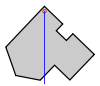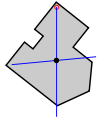(a) (b) (c)

This method can be extended (in theory) to concave shapes where the centroid lies outside the shape, and to solids (of uniform density), but the positions of the plumb lines need to be recorded by means other than drawing.

### Balancing Method

For convex two-dimensional shapes, the centroid can be found by balancing the shape on a smaller shape, such as the top of a narrow cylinder. The centroid occurs somewhere within the range of contact between the two shapes. In principle, progressively narrower cylinders can be used to find the centroid to arbitrary accuracy. In practice air currents make this unfeasible. However, by marking the overlap range from multiple balances, one can achieve a considerable level of accuracy.

### Of a finite set of points

The centroid of a finite set of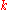pointsin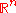is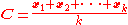### By geometric decomposition

The centroid of a plane figure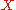can be computed by dividing it into a finite number of simpler figures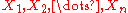, computing the centroid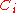and area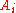of each part, and then computing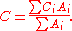Holes in the figure, overlaps between the parts, or parts that extend outside the figure can all be handled using negative areas. Namely, the measuresshould be taken with positive and negative signs in such a way that the sum of the signs offor all parts that enclose a given point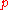is 1 ifbelongs to, and 0 otherwise.

For example, the figure below (a) is easily divided into a square and a triangle, both with positive area; and a circular hole, with negative area (b).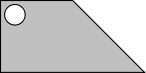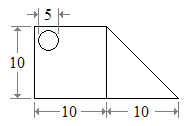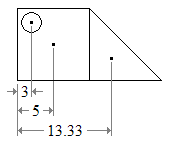(a) (b) (c)

The centroid of each part can be found in any list of centroids of simple shapes (c). Then the centroid of the figure is the weighted average of the three points. The horizontal position of the centroid, from the left edge of the figure is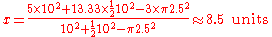The same formula holds for any three-dimensional objects, except that eachshould be the volume of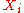, rather than its area. It also holds for any subset of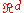, for any dimension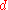, with the areas replaced by the-dimensional measure
Measure (mathematics)
In mathematical analysis, a measure on a set is a systematic way to assign to each suitable subset a number, intuitively interpreted as the size of the subset. In this sense, a measure is a generalization of the concepts of length, area, and volume...

s of the parts.

### By integral formula

The centroid of a subset X of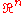can also be computed by the integral
Integral
Integration is an important concept in mathematics and, together with its inverse, differentiation, is one of the two main operations in calculus...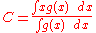where the integral is taken over the whole space, and g is the characteristic function of the subset, which is 1 inside X and 0 outside it. Note that the denominator is simply the measure
Measure (mathematics)
In mathematical analysis, a measure on a set is a systematic way to assign to each suitable subset a number, intuitively interpreted as the size of the subset. In this sense, a measure is a generalization of the concepts of length, area, and volume...

of the set X (However, this formula cannot be applied if the set X has zero measure, or if either integral diverges.)

Another formula for the centroid is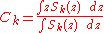where Ck is the kth coordinate of C, and Sk(z) is the measure of the intersection of X with the hyperplane defined by the equation xk = z. Again, the denominator is simply the measure of X.

For a plane figure, in particular, the barycenter coordinates are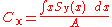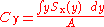where A is the area of the figure X; Sy(x) is the length of the intersection of X with the vertical line at abscissa
Abscissa
In mathematics, abscissa refers to that element of an ordered pair which is plotted on the horizontal axis of a two-dimensional Cartesian coordinate system, as opposed to the ordinate...

x; and Sx(y) is the analogous quantity for the swapped axes.

### Of an L-shaped object

This is a method of determining the centroid of an L-shaped object.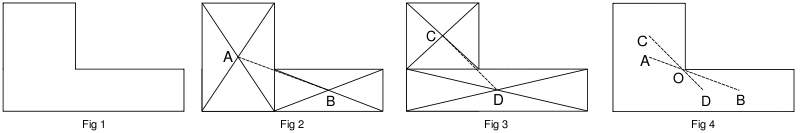1. Divide the shape into two rectangles, as shown in fig 2. Find the centroids of these two rectangles by drawing the diagonals. Draw a line joining the centroids. The centroid of the shape must lie on this line AB.
2. Divide the shape into two other rectangles, as shown in fig 3. Find the centroids of these two rectangles by drawing the diagonals. Draw a line joining the centroids. The centroid of the L-shape must lie on this line CD.
3. As the centroid of the shape must lie along AB and also along CD, it is obvious that it is at the intersection of these two lines, at O. The point O might not lie inside the L-shaped object.

### Of triangle and tetrahedron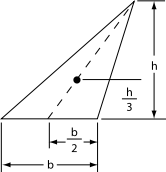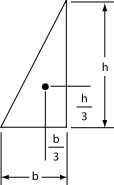The centroid of a triangle
Triangle
A triangle is one of the basic shapes of geometry: a polygon with three corners or vertices and three sides or edges which are line segments. A triangle with vertices A, B, and C is denoted ....

is the point of intersection of its medians
Median (geometry)
In geometry, a median of a triangle is a line segment joining a vertex to the midpoint of the opposing side. Every triangle has exactly three medians; one running from each vertex to the opposite side...

(the lines joining each vertex
Vertex (geometry)
In geometry, a vertex is a special kind of point that describes the corners or intersections of geometric shapes.-Of an angle:...

with the midpoint of the opposite side). The centroid divides each of the medians in the ratio
Ratio
In mathematics, a ratio is a relationship between two numbers of the same kind , usually expressed as "a to b" or a:b, sometimes expressed arithmetically as a dimensionless quotient of the two which explicitly indicates how many times the first number contains the second In mathematics, a ratio is...

2:1, which is to say it is located ⅓ of the perpendicular distance between each side and the opposing point (see figures at right). Its Cartesian coordinates are the means
Arithmetic mean
In mathematics and statistics, the arithmetic mean, often referred to as simply the mean or average when the context is clear, is a method to derive the central tendency of a sample space...

of the coordinates of the three vertices. That is, if the three vertices are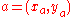,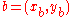, and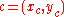, then the centroid is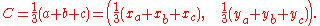The centroid is therefore at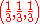in barycentric coordinates
Barycentric coordinates (mathematics)
In geometry, the barycentric coordinate system is a coordinate system in which the location of a point is specified as the center of mass, or barycenter, of masses placed at the vertices of a simplex . Barycentric coordinates are a form of homogeneous coordinates...

.

The centroid is also the physical center of mass if the triangle is made from a uniform sheet of material; or if all the mass is concentrated at the three vertices, and evenly divided among them. On the other hand, if the mass is distributed along the triangle's perimeter, with uniform linear density
Linear density
Linear density, linear mass density or linear mass is a measure of mass per unit of length, and it is a characteristic of strings or other one-dimensional objects. The SI unit of linear density is the kilogram per metre...

, the center of mass may not coincide with the geometric centroid.

The area of the triangle is 1.5 times the length of any side times the perpendicular distance from the side to the centroid.

Similar results hold for a tetrahedron
Tetrahedron
In geometry, a tetrahedron is a polyhedron composed of four triangular faces, three of which meet at each vertex. A regular tetrahedron is one in which the four triangles are regular, or "equilateral", and is one of the Platonic solids...

: its centroid is the intersection of all line segments that connect each vertex to the centroid of the opposite face. These line segments are divided by the centroid in the ratio 3:1. The result generalizes to any n-dimensional simplex
Simplex
In geometry, a simplex is a generalization of the notion of a triangle or tetrahedron to arbitrary dimension. Specifically, an n-simplex is an n-dimensional polytope which is the convex hull of its n + 1 vertices. For example, a 2-simplex is a triangle, a 3-simplex is a tetrahedron,...

in the obvious way. If the set of vertices of a simplex is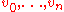, then considering the vertices as vectors, the centroid is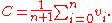The geometric centroid coincides with the center of mass if the mass is uniformly distributed over the whole simplex, or concentrated at the vertices as n equal masses.

The isogonal conjugate
Isogonal conjugate
In geometry, the isogonal conjugate of a point P with respect to a triangle ABC is constructed by reflecting the lines PA, PB, and PC about the angle bisectors of A, B, and C. These three reflected lines concur at the isogonal conjugate of P...

of a triangle's centroid is its symmedian point
Symmedian
Symmedians are three particular geometrical lines associated with every triangle. They are constructed by taking a median of the triangle , and reflecting the line over the corresponding angle bisector...

.

### Centroid of polygon

The centroid of a non-self-intersecting closed polygon defined by n vertices (x0,y0), (x1,y1), ..., (xn−1,yn−1) is the point (Cx, Cy), where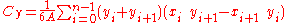and where A is the polygon's signed area,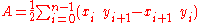In these formulas, the vertices are assumed to be numbered in order of their occurrence along the polygon's perimeter, and the vertex ( xn , yn ) is assumed to be the same as ( x0 , y0 ). Note that if the points are numbered in clockwise order the area A, computed as above, will have a negative sign; but the centroid coordinates will be correct even in this case.

### Centroid of cone or pyramid

The centroid of a cone or pyramid is located on the line segment that connects the apex
Apex (geometry)
In geometry, an apex is the vertex which is in some sense the highest of the figure to which it belongs.*In an isosceles triangle, the apex is the vertex where the two sides of equal length meet, opposite the unequal third side....

to the centroid of the base. For a solid cone or pyramid, the centroid is 1/4 the distance from the base to the apex. For a cone or pyramid that is just a shell (hollow) with no base, the centroid is 1/3 the distance from the base plane to the apex.

## See also

• List of centroids
• Fréchet mean
Fréchet mean
The Fréchet mean , is the point, x, that minimizes the Fréchet function, in cases where such a unique minimizer exists. The value at a point p, of the Fréchet function associated to a random point X on a complete metric space is the expected squared distance from p to X...

• Pappus's centroid theorem
Pappus's centroid theorem
In mathematics, Pappus' centroid theorem is either of two related theorems dealing with the surface areas and volumes of surfaces and solids of revolution....

• K-means algorithm
K-means algorithm
In statistics and data mining, k-means clustering is a method of cluster analysis which aims to partition n observations into k clusters in which each observation belongs to the cluster with the nearest mean...

• Triangle center
Triangle center
In geometry a triangle center is a point in the plane that is in some sense a center of a triangle akin to the centers of squares and circles. For example the centroid, circumcenter, incenter and orthocenter were familiar to the ancient Greeks, and can be obtained by simple constructions. Each of...

• Chebyshev center
Chebyshev center
In geometry, the Chebyshev center of a bounded set Q having non-empty interior is the center of the minimal-radius ball enclosing the entire set Q, or, alternatively, the center of largest inscribed ball of Q ....

## External links

The source of this article is wikipedia, the free encyclopedia.  The text of this article is licensed under the GFDL.